International Journal of Biomedical Science and Engineering
Volume 3, Issue 2, April 2015, Pages: 25-33

Coherence Function in Noisy Linear System

Cecil W. Thomas

Biomedical Engineering Department, Saint Louis University, St Louis, MO USA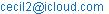Cecil W. Thomas. Coherence Function in Noisy Linear System. International Journal of Biomedical Science and Engineering. Vol. 3, No. 2, 2015, pp. 25-33. doi: 10.11648/j.ijbse.20150302.13

Abstract: The coherence function provides a measure of spectral similarity of two signals, but measurement noise decreases the values of measured coherence. When the two signals are the input and output of a linear system, any system noise also decreases the measured coherence values. In digital computations, useful coherence values require some degree of averaging to increase the degrees of freedom to more than two. These fundamental issues are presented with application to system input-output coherence and two random signals with a common component. Finally, estimated coherence of the two random signals, with varying degrees of freedom, are shown with empirical adjustments that can improve the estimate of coherence. Coherence has a wide range of biomedical applications, but this article focuses on the fundamental properties of the coherence function.

Keywords: Coherence, Noise, Similarity, Degrees of Freedom, Linear System

1. Introduction

The coherence function is a frequency domain measure of the "likeness" of two functions or signals. Qualitatively, it is a correlation coefficient vs. frequency, although the analogy should not be pursued in any strict sense. The correlation coefficient is a normalized covariance, while the coherence function is a normalized cross-power spectrum. The correlation coefficient is a scalar measure of the similarity of the overall shapes of two functions; the coherence function is a vector measure of the similarity in frequency content of two signals. For two identical signals, the correlation coefficient is unity and the coherence function is unity. Two random uncorrelated signals yield a correlation coefficient and coherence function of zero. However, unlike the coherence function, the correlation coefficient is sensitive to phase. Two sinusoids at the same frequency have a correlation coefficient that varies from -1 to +1 as the relative phase of the sinusoids varies from zero to π. As illustrated later, the coherence function is insensitive to phase.

Coherence is a normalized cross-power spectrum that can be used as a measure of the spectral similarity of two signals, or as a measure of the degree to which two signals have a common source. Coherence has been used in modeling linear systems [1-2], estimating system time delay [3-6], and estimating system nonlinearities [7-11]. Its computation [12-19] is based on the standard Fourier Transform and correlation methods, with some additional considerations for discrete computation and various biases in the estimated values [20-25]. When frequency resolution in the estimate is limited in time-varying or transient cases, coherence can still be useful with certain nonstationary processes .

Coherence is insensitive to phase, and it is amplitude normalized, but the coherence is sensitive to uncorrelated noise and system nonlinearities because both introduce disparities between the two signal spectra. Within a frequency band, coherence is reduced by additive noise that is uncorrelated in the two signals, and thus it can be a useful vector measure of signal-to-noise ratio. For example, the coherence of input and output of a linear system can show the frequency bands where signal-to-noise ratio is sufficiently high for useful calculations in system identification.

The coherence function of two signals x(t) and y(t) is defined by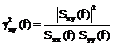(1)

where Sxy(f) is the cross-power spectrum of x(t) and y(t), Sxx(f) is the auto-power spectrum of x(t), and Syy(f) is the auto-power spectrum of y(t). The cross-power spectrum and the auto-power spectra can be computed from the Fourier Transform of signals x and y.

This tutorial focuses on the basic properties of the coherence of two signals in the presence of noise, and the computation of coherence in continuous and discrete systems. The coherence function is applied to the input and output of a system, to examine the effects of measurement noise and system noise. Generally, the coherence function is reduced by noise that is not common to both input and output of the system.

The digital computation of the coherence function will be considered along with the pitfalls and approximations in the discrete coherence function computation. Two methods for digital computation will be discussed. One method involves segmenting the time domain function into several subsegments, computing the transform of each subsegment, and then combining the separate results in the frequency domain. The second method transforms the entire time domain signal, and then averages over frequency bands.

Finally, two Gaussian white noise functions are used to demonstrate the effect of the number of degrees of freedom on estimates of the coherence function. The quality of the coherence estimate can be assessed in two ways. Measured coherence values can be adjusted using an empirical expression. The other quality measure is based on confidence intervals as defined in [27, 28].

A separate paper addresses the effects of system nonlinearity on the input-output coherence.

2. Coherence in Linear Noise-Free System

For a linear time-invariant system, the input x(t) and the output y(t) are related by

y(t) = x(t) * h(t)                (2)

and

Y(f) = X(f) H(f)                (3)

where h(t) is the system impulse response, H(f) is the system transfer function, and * denotes convolution. The auto-power spectra of x(t) and y(t) are

Sxx(f) = X(f) X*(f)              (4)

and

Syy(f) = Y(f) Y*(f)              (5)

where the superscript * denotes the complex conjugate. The cross-power spectrum of x(t) and y(t) is

Sxy(f) = X*(f) Y(f)             (6)

Using Equations (4), (5), and (6), the coherence function can be computed by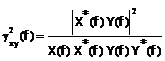(7)

The coherence function can also be expressed in terms of the system transfer function. In general, the transfer function is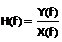(8)

Multiplying numerator and denominator by the complex conjugate of X(f), Equation (8) becomes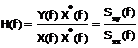(9)

The squared magnitude of the transfer function is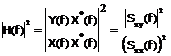(10)

Multiplying numerator and denominator by Syyf),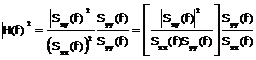(11)

But the factor in brackets is the coherence function, as in Equation (1). Thus,(12)

Solving for the coherence function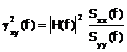(13)

From Equations (4) and (5),(14)

Substituting Equation (14) into Equation (13)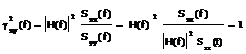(15)

The unity coherence value can be rationalized by the following. In a linear noise-free system, the frequency content of the output is the same as the frequency content of the input; only the magnitudes and phases of the frequency components are altered by the system. Since the coherence function is an amplitude-normalized phase-insensitive measure of the common components, the coherence function for the input and output of a linear noise-free system is unity, indicating maximum or complete coherence.

3. Coherence in Linear System with Noise

When random noise is introduced, due to measurement error, circuit thermal noise, etc, the input and output of the linear system will have frequency components that are not common to both. The coherence function will be reduced by the noise as illustrated by the following.

The linear system with impulse response h(t) and input u(t), has an output v(t). Suppose that our measurements of u(t) and v(t) introduce noise, resulting in the observed x(t) and y(t) as the input and output, where

x(t) = u(t) + n1(t)           (16)

and

y(t) = v(t) + n2(t)           (17)

as illustrated in Figure 1.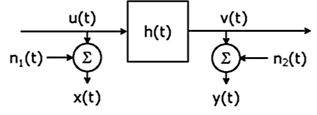Figure 1. System with additive noise in measurement of input and output.

Assuming the noise is uncorrelated with the input and output, the power spectra of the observed signals are

Sxx(f) = Suu(f) + N1(f)            (18)

and

Syy(f) = Svv(f) + N2(f)            (19)

where N1(f) and N2(f) are the power spectra of the noise at the input and output, respectively. If the two noise functions are random and uncorrelated, the cross-power spectrum is not affected by the noise, so that

Sxy(f) = Suv(f)             (20)

and the coherence function is(21)

Expanding the denominator, and dividing numerator and denominator by Suu(f) Svv(f),(22)

When the noise spectra are both zero, the denominator in Equation (22) goes to unity, indicating that the measured coherence is the actual coherence of the input and output. However, when either noise source is non-zero, the denominator is greater than one, and the measured coherence is less that the actual coherence of u and v. Therefore, in the presence of random uncorrelated noise, the measured coherence is(23)

Equality holds when the noise is zero, and noise in either input or output will reduce the measured coherence.

4. Digital Computation of Coherence

The coherence function computed digitally (on sampled data) using Equation (1) is unity at all frequencies for any two functions x(t) and y(t). At a given discrete frequency fk, the signals have the form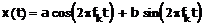(24a)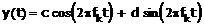(24b)

where t = nT, and T is the sampling interval. In the discrete transform, the coefficient at each discrete frequency fk has the form(25a)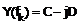(25b)

where A=sa, B=sb, C=sc, D=sd, and s is a software-dependent scaling constant that is typically 0.5.

The coherence is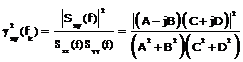(26a)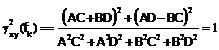(26b)

Notice that the coherence is unity for all frequencies, for any signals x and y, and for noiseless or noisy signals. Also, scaling one or both signals has no effect, because the scaling factors in numerator are cancelled by the factors in the denominator. In other words, the result is not useful.

When B=C=0, Equation (26) gives the coherence of a sine and cosine, which is unity. Note than this result differs from a computed correlation coefficient which would be zero. Both the scalar correlation coefficient and vector coherence are measures of the similarity of two signals. However, the correlation coefficient is sensitive to phase (as in sine vs cosine), but coherence is insensitive to phase.

When computed digitally, the coherence function must be defined as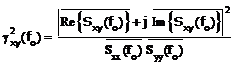(27)

where the bar represents averaging over M elementary bandwidths, ie, over M discrete frequencies for which there are coefficients in the transform in the neighborhood of fo.

In the analog case a coherence value would represent an average over an infinite number of frequencies within a band. The digital case must approximate the analog case by averaging over a finite number of the discrete frequencies in the band. With no such averaging, ie, with two degrees of freedom (from squaring the real part and the imaginary part), the digitally computed coherence is always unity, as demonstrated above. How much averaging should be done, ie, what 2M degrees of freedom are required to get a good measure of coherence, will be discussed later. Notice that the division by M in each individual average is not necessary for the calculation of coherence using Equation (27), because the division in the numerator cancels the division in the denominator. Therefore, the average can be implemented as a simple summation.

Equation (27) shows that the coherence computed at a single frequency is always unity. While the problem is described for discrete computation, single-frequency (monochromatic) coherence occurs whenever the time-domain signal is periodic over all time. The signal could be discrete (as in fft-type computation) or it could be analog (as in a Fourier Series computation). In these cases, the computation of coherence at a single frequency is not influenced by energy at any other frequency. However, when an analog time-domain signal has finite duration, the spectral window introduces an averaging among neighboring frequency components.

In the continuous case, with signals of finite duration, a finite range on frequency will contain an infinite number of components, and the coherence function may be less than unity. In discrete computations, the spectral window plays the same role, except for the case where a rectangular window contains exactly an integer number of cycles of all frequency components in the signal. The smoothing (averaging) over M elementary bandwidths introduced in Equation (27) expands the number of frequency components in a finite frequency region, and the coherence may also be less than unity.

4.1. Coherence of Two Sampled Signals

In the following example, the coherence function will be computed assuming that P seconds of both functions are sampled, and that the FFT is used to transform the entire P seconds segment of data. As an example, let

x(t) = A1 cos[2π3fot]+A2 sin[2π3fot]+A3 cos[2π4fot]  (28a)

and

y(t)=B1 cos[2π3fot]+B2 sin[2π3fot] + B3 cos[2π4fot]  (28b)

With no averaging (ie, with 2 degrees of freedom), the coherence function at f = 3fo and f = 4fo would be unity. However, using Equation (27), we can compute the coherence function by averaging over two elementary bands to get 2M = 4 degrees of freedom. Therefore, the function at f = f1 = [3fo + 4fo] / 2 is computed as follows.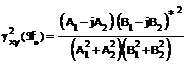(29)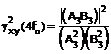(30)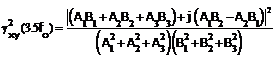(31)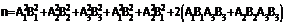(32)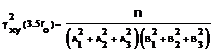(33)

If Ai = Bi = 1 for i = 1, 2, 3, the two signals are identical and the coherence function is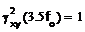(34)

Similarly, if Ai = Bi = any constant, the coherence is unity.

More generally, if Ai = Ki Bi, the coherence is unity only when the constants Ki are all equal. In that special case, the spectra of x and y have the same shape - only the relative amplitude scaling is changed. In contrast, the two spectra have different shapes when the constants are different, and different shapes result in coherence values less than unity. For example, let Bi = 1, and

A1 = 2 B1                (35a)

A2 = 3 B2                (35b)

A3 = 5 B3                (35c)

Then the coherence in Equation (33) has a value of 0.886.

To further illustrate the relation between spectral shape differences and the coherence function, let all the Ai = 1, for all i, and Bi = 1 for all except one i. If we vary any of the Bi values, the resulting coherence is shown in Figure (2).

Figure (2) represents the coherence when B1 is varied, but the same results are obtained by varying B2 with B1 = 1. In the frequency domain, B1 represents the real part at a frequency 3fo and B2 represents the imaginary part at the same frequency. Since coherence is insensitive to phase, varying either the real part or the imaginary part has the same effect. Notice that for large absolute values of B1 or B2, the coherence value approaches 0.667. Overall, the coherence values vary between 0.5 and 1.0.

A second example is illustrated in Figure (3), by varying B1 with different values of the coefficients in Equation (28). Notice that the coherence varies between zero and unity, and coherence approaches 0.5 for large values of B1.

4.2. Coherence by Segmentation

Instead for transforming the entire length of the functions x(t) and y(t), consider subdividing the data and transforming each segment separately. Then, the coherence is computed by averaging over the transformed segments. This segmentation

method will now be examined and compared with the coherence obtained in the previous section.

The two methods in question may be described as follows. In Method A, used in the previous section, the procedure is as follows.

Method A. Smooth in Frequency

1.       transform P seconds of x(t) and y(t) using the FFT,

2.       using the transformed data from step 1, calculate Sxx(f), Syy(f), and Sxy(f),

3. smooth the three spectra from step 2, where the smoothing is over M elementary bandwidths to obtain the coherence with 2M degrees of freedom.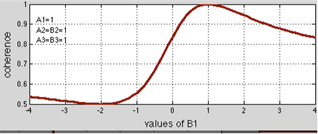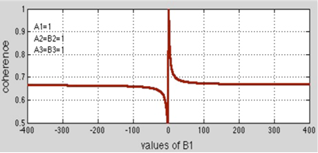Figure 2. Coherence from Equation (33) when all As and Bs are unity except for B1. The coherence varies from 0.5 to 1.0, and converges to 0.667 at large values of B1.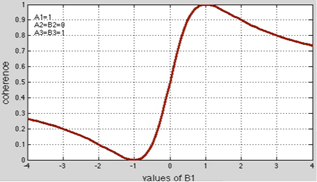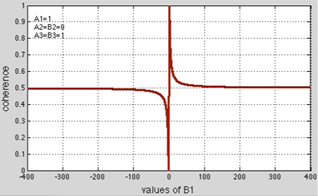Figure 3. Coherence from Equation (33) as in Figure 2, but A2 = B2 = 0 instead of unity. The resulting coherence varies from 0 to 1.0, and converges to 0.5 at large values of B1.

Method B, Segmentation in Time

1. transform each of the M segments of x(t) and y(t), where each segment is P/M seconds,

2. using the transformed data from step 1, compute Sxx(f), Syy(f), Sxy(f) for each of the M segments,

3. average the corresponding spectra from the M segments, to obtain the coherence with 2M degrees of freedom.

In Method A, 2M degrees of freedom are achieved by averaging over M elementary bandwidths, while in Method B, averaging M segments also achieves the same 2M degrees of freedom.

In a global sense, the two methods give the same results for signals like random noise. The other extreme would be a sinusoid (even with frequency modulation), where the segmentation method could result in segments containing only a fraction of a cycle of the sinusoid. Other deterministic components could also be affected by the segmentation, but in most cases, the two methods should produce comparable results.

The two methods have subtle differences, so that the coherence results of the two methods are not exactly equivalent. Consider a P-seconds signal, and M=3. In Methods A, the frequencies before averaging are at intervals of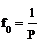(36)

After averaging (with M=3), the frequencies are at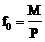(37)

The first frequency (after f=0) is at the average of f0, 2f0, and 3fo. The center frequency is 2fo which becomes the location of the first coherence value. Then, the frequencies (after averaging) are(38)

Notice that the first frequency is at 2f0, then the spacing is at intervals of 3fo.

In Method B, the segments have durations of P/M seconds, so the fundamental frequency and the frequency spacing are given by(39)

Then, the frequencies (after averaging over segments) are at(40)

Notice that the first frequency and the frequency spacing are both equal to 3fo.

Comparing the frequencies in Equations (38) and (40), the frequency spacing is the same for both methods. However, the first frequency (lowest above f=0) is different in the two methods because of the way that the averaging is accomplished. In most cases, this difference is trivial, but it is a subtle difference in the two methods.

The more significant difference can be caused by segmentation. For example, if three cycles of the cosine are split into three segments, each segment is still a cosine at a single frequency. However, if the number of segments were increased, each segment would be a fraction of a cycle, and leakage would dominate the computed spectrum for each segment and in the final result. Therefore, for deterministic functions, the segmentation method is limited by the length of signal available and the frequency content of that signal. An excessive number of segments will degrade the coherence estimate by decreasing the frequency resolution.

One solution is to use overlapping segments as advocated in . The recommended overlap is 50%. The overlapping has the advantage of increasing both the time duration of each segment and the number of samples per segment. However, overlap of more than about 50% leads to highly correlated coherence estimates and additional computation. The same argument holds for deterministic signals where overlapping segments can lead to better frequency resolution and less leakage at low frequencies.

5. Coherence of Two Random Signals

In previous sections, the coherence function was computed for sinusoidal signals. Now consider the signals

x(t) = z(t) + a n1(t)          (41a)

and

y(t) = z(t) + b n2(t)          (41b)

where z(t), n1(t), and n2(t) are Gaussian noise functions with unity variance and zero mean. If we consider z(t) to be the input to a unity gain noiseless system, a n1(t) to be the noise in the input measurement, and b n2(t) to be the noise in the output measurement, the coherence function can be calculated by Equation (22). Then for this random case,

(42)

The variance of z is unity, Szz(f) = 1, and the noise variance is unity, so Equation (42) reduces to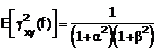(43)

Figure 4 shows the coherence when one noise amplitude is zero, and when both noise components have equal amplitude.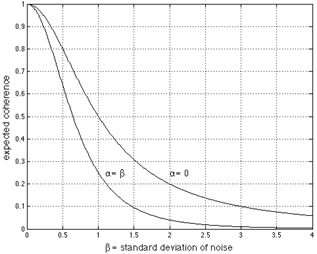Figure 4. Expected values of coherence for different noise levels, from Equation (43).

6. Coherence & Degrees of Freedom

The expected values of the coherence function, as given by Equation (43) and Figure 4, do not include the effect of the degrees of freedom in the digital calculation. It was shown earlier that for two degrees of freedom, computed coherence was always unity regardless of the true coherence. As a matter of notation, the digitally computed coherence will be called the "sample coherence" to distinguish it from the expected coherence given by Equation (43).

To illustrate the relationship between the sample coherence and the degrees of freedom, the functions in Equations (41) are simulated using Gaussian white noise in z(t), n1(t), and n2(t) where the three signals are mutually uncorrelated, and each has zero mean and unity variance. Then the signals x(t) and y(t) have a common component, namely z(t). They have additive uncorrelated noise whose variances are determined by a and b. As in Figure (4), consider two cases, one with a= 0, and the other with a= b.

Using 4096 samples of x(t) and y(t), the sample coherence was computed for different degrees of freedom, and plotted in Figure (5) for a The horizontal dotted lines show the expected coherence from Equation (46). Figure (6) show the results when a b

In both Figure (5) and Figure (6), the coherence is shown for degrees of freedom (dof) starting at 4. For 2 degrees of freedom, all curves go to a coherence of 1.0. Notice that the sample coherence and the expected coherence differ significantly for lower dof. At higher values of dof, the curves approach the expected values, and the convergence is slower at larger values of noise (ie, values of a and b). This makes sense because higher noise levels require more averaging or smoothing, and in this case, more degrees of freedom equates to more averaging.

The coherence values are higher in Figure 5 (than in Figure 6). This is also intuitive, because x(t) has no noise. Thus, x(t) and y(t) are more similar, since they have the common component z(t) and only one noise component to decease their similarity. When the noise in x(t) is non-zero, ie, when a is nonzero, the coherence values are lower, as seen in Figure 6.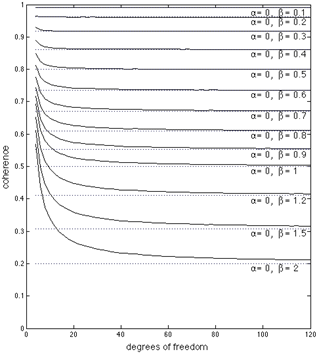Figure 5. Sample coherence (solid curves) and expected coherence (dotted lines) for a range of degrees of freedom, and with noise only in y(t), ie, with a= 0.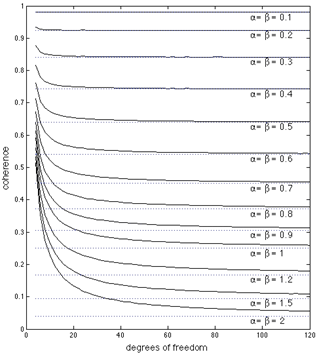Figure 6. Sample coherence (solid curves) and expected coherence (dotted lines) for different levels of noise and for a range of degrees of freedom. In this case, the noise level in x(t) and y(t) are the same, ie, a=b

7. Estimating Expected Coherence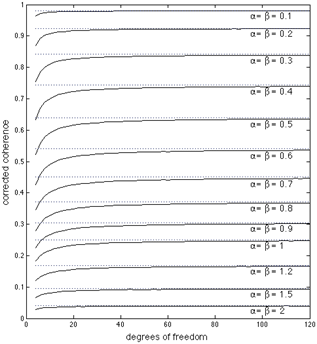Figure 7. Coherence after applying the empirical correction in Equation (44) to the data in Figure (6).

The relationship between the sample coherence and the expected value of coherence can be expressed by the empirical equation where the subscript E indicates expected value, and the subscript s indicates sample values. Let's apply the correction to the earlier example in Equations (41) with computed sample coherence in Figures (6). The results are shown in Figures (7).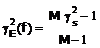(44)

The empirical equation in Equation (44) over-compensates for lower values of degrees of freedom. Compare Figures 6 and 7. The uncorrected coherence values are actually better for high coherence values (and low dof). However, the corrected values are significantly better at lower coherence values, especially at low dof.

The approximation in Equation (44) is crude and can be improved by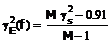(45)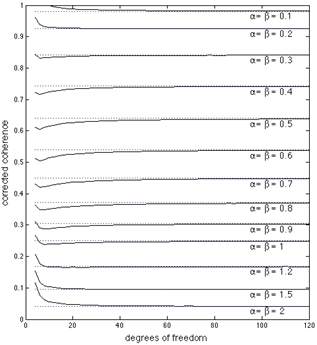Figure 8. Corrected coherence values from applying Equation (45) to the sample coherence in Figure (6).

Applying this modified correction to the sample coherence in Figure (6), the resulting coherence is shown in figure (8). Comparing the corrected values in Figure (7) and Figure (8), the modified correction formula in Equation (45) appears to have some advantage.

It should be noted that the coherence values, corrected and uncorrected, represent averaged data. In a single computation of sample coherence, the actual coherence is affected by noise and degrees of freedom. The curves in the figures can be used as guides, or even calibration curves, but in any single case, the sample coherence should be considered as an estimate of the actual coherence. In many applications, the relative coherence may be the desired measure, and the uncorrected sample coherence is sufficient.

The last example illustrates, as expected, that coherence is deceased by noise in either signal. Additionally, the sample coherence and expected coherence differ by an amount that varies with the coherence values and the degrees of freedom. The sample coherence can be corrected to obtain values that are closer to the expected coherence.

More generally, the last example might represent two measurements x and y that originate from a common source. For example, suppose the signal z(t) is a source of normal or abnormal activity in neural tissue. Then, x(t) and y(t) might be signals from two different electrodes at two locations that are remote from the center of the activity z(t). Each of the two measurements are degraded by noise, and a higher level of noise in either recording, leads to lower values of sample coherence.

We assume that both x(t) and y(t) are linearly related to z(t). In linear cases, the amplitude scaling does not affect the sample coherence. In the absence of noise, the sample coherence values would be unity. Even if the tissue path between z(t) and x(t) is a different linear function than for the tissue path from z(t) to y(t), the two linear functions would introduce only scaling factors to x(t) and y(t), and probably some phase shifts. However, the sample coherence is insensitivity to both the scaling factors and the phase shifts. Thus, the coherence in these linear cases is degraded by noise.

8. Conclusion

The properties of the coherence function have been presented with emphasis on its application to the input and output of a linear system. Since the coherence function is a normalized measure of the spectral similarity of two signals, the coherence of the input and output of a linear noise-free system is unity. However, additive noise in the measurement of either the input or the output of a linear system will reduce the coherence function. In general, any noise component not common to both input and output will reduce the coherence.

For random signals such as Gaussian noise, the coherence function may be computed by either of two methods: (A) transform the entire length of data and smooth over M elementary bandwidths to get 2M degrees of freedom, or (B) divide the data into segments which are transformed individually, then combine the transformed results to get 2M degrees of freedom. These two methods yield equivalent results, but the coherence values are at slightly different discrete frequencies. For deterministic signals, the segmentation in Method B increases the leakage, but overlapping segments can partially compensate. An overlap of about 50% may be useful.

The sample coherence approaches the expected coherence as the number of degrees of freedom is increased. At lower degrees of freedom, the coherence estimate can be improved by a correction expression using the sample coherence and the degrees of freedom.

References

1. Bendat J and Piersol A. Random Data, Analysis and Measurement Procedures. John Wiley and Sons, New York, 1986.
2. Cadzow A and Solomon OM. Linear modeling and the coherence function. IEEE Trans. Acoust, Speech, Signal Processing, vol. 35, no. 1, pp. 19-28, 1987.
3. Hannan EJ and Thomson PJ. Delay estimation and the estimation of coherence and phase. IEEE Trans. Acoust, Speech, Signal Processing, vol. 29, no. 3, pp. 485-490, 1981.
4. Chan YT and Miskowisz RK. Estimation of time delay with ARMA models. IEEE Trans. Acoust, Speech, Signal Processing, vol. 32, no.2, pp. 295-303, 1984.
5. Carter GC. Coherence and time delay estimation. Proc. IEEE, vol. 75, no. 2, pp. 236-255, 1987.
6. Carter G. Coherence and time delay estimation. C. Chen, Signal Processing Handbook, Marcel Dekker, New York, 1988.
7. Kim YC, Wong WF, Powers EJ, Roth JR. Extension of the coherence function to quadratic models. Proc. IEEE, vol. 67, no. 3, pp. 428-429, 1979.
8. Kim KI. On measuring the system coherency of quadratically nonlinear systems. IEEE Trans. Signal Processing, vol. 39, no. 1, pp. 212-214, 1991.
9. Maki BE. Interpretation of the coherence function when using pseudorandom inputs to identify nonlinear systems. IEEE Trans. Biomed. Engr, vol. 33, no. 8, pp. 775-779, 1986.
10. Maki BE. Addendum to 'Interpretation of the coherence function when using pseudorandom inputs to identify nonlinear systems'. IEEE Trans. Biomed. Engr, vol. 35, no. 4, pp. 279-280, 1988.
11. Cho YS, Kim SB, Hixson EL, Powers EJ. A Digital Technique to Estimate Second-Order Distortion Using Higher Order Coherence Spectra. IEEE Trans. Signal Processing, vol. 40, no. 5, pp. 1029-1040, 1992.
12. Benignus VA. Estimation of the coherence spectrum and its confidence interval using the fast Fourier transform. IEEE Trans. Audio Electroacoustics, vol. 17, no. 2, pp.145-150, 1969.
13. Carter GC, Knapp CH, Nuttall A. Estimation of the magnitude squared coherence function via overlapped fast Fourier transform Processing. IEEE Trans. on Audio and Acoustics, vol. 21, no. 4, pp. 337-344, 1973.
14. Carter GC and Knapp CH. Coherence and Its Estimation via the Partitioned Modified Chirp-Z Transform. IEEE Trans. Acoust, Speech, Signal Processing, vol. 23, no. 3, pp. 257-264, 1975.
15. Foster M and Guinzy NJ. The coefficient of coherence: its estimation and its use in geophysical data processing. Geophysics, vol. 32, no. 4, pp. 602-616, 1967.
16. Lee PF. An algorithm for computing the cumulative distribution function for magnitude-squared coherence estimates. IEEE Trans. Acoust, Speech, Signal Processing, vol. 29, no. 2, pp. 117-119, 1973.
17. Nuttall AH and Carter GC. An approximation to the cumulative distribution function of the magnitude-squared coherence estimate. IEEE Trans. Acoust, Speech, Signal Processing, vol. 29, no. 4, pp. 932-934, 1981.
18. Walden AT. Maximum likelihood estimation of magnitude-squared multiple and ordinary coherence. Signal Processing, vol. 19, no. 1, pp. 75-83, 1990.
19. Youn DH, Ahmed N, Carter GC. Magnitude-squared coherence function estimation and adaptive approach. IEEE Trans. Acoust, Speech, Signal Processing, vol. 31, no. 1, pp. 137-142, 1983.
20. Carter GC. Bias in magnitude coherence estimation due to misalignment. IEEE Trans. Acoust, Speech, Signal Processing, vol. 28, no. 1, pp. 97-99, 1980.
21. Evensen HA and Trethewey MW. Bias errors in estimating frequency response and coherence functions from truncated transient signals. Journal of Sound and Vibration, vol. 145, no. 1, pp. 1-16, 1991.
22. Kroenert JT. Some comments on bias/misalignment effects in the magnitude squared coherence estimate. IEEE Trans. Acoust, Speech, Signal Processing, vol. 30, no. 3, pp. 511-513, 1982.
23. Nuttall AH and Carter GC. Bias of the estimate of magnitude-squared coherence. IEEE Trans. Acoust, Speech, Signal Processing, vol. 24, no. 6, pp. 582-583, 1976.
24. Stearns SD. Tests of coherence unbiasing methods. IEEE Trans. Acoust, Speech, Signal Processing, vol. 29, no. 2, pp. 321-323, 1981
25. Scannell EH and Carter GC. Confidence bounds for magnitude-squared coherence estimates. IEEE Trans. Acoust, Speech, Signal Processing, vol. 26, no.5, pp. 475-477, 1978.
26. Gardner WA, On the Spectral Coherence of Nonstationary Processes. IEEE Trans. Signal Processing, vol 39, no 2, pp424-431, 1991

Article ToolsAbstractPDF(825K)